# How to calculate percentage in Excel - formula examples

In this tutorial, you will lean a quick way to calculate percentages in Excel, find the basic percentage formula and a few more formulas for calculating percentage increase, percent of total and more.

Calculating percentage is useful in many areas of life, whether it is restaurant tipping, reseller commission, your income tax or interest rate. Say, you've been lucky enough to get a 25% off promotion code on a new plasma TV. Is this a good deal? And how much will you eventually have to pay?

In this tutorial, we are going to explore a few techniques that will help you efficiently calculate percent in Excel and learn the basic percentage formulas that will take the guesswork out of your calculations.

## Percentage basics

The term "per cent" is derived from the Latin per centum, meaning "by the hundred". As you probably remember from high school math class, a percentage is a fraction of 100 that is calculated by dividing the numerator by the denominator and multiplying the result by 100.

The basic percentage formula is as follows:

(Part/Whole)*100 = Percentage

For example, if you had 20 apples and you gave 5 to your friends, how much did you give, percentage wise? By performing a simple calculation `=5/20*100` you get the answer - 25%.

This is how you normally calculate percentages in school and everyday life. Computing percentage in Microsoft Excel is even easier since Excel performs some operations for you automatically, in the background.

Regrettably, there is no universal Excel formula for percentage that would cover all possible scenarios. If you ask someone "Which percent formula do I use to get the result I want?", most likely, you will get an answer like "Well, that depends on what exactly result you want to achieve."

So, let me show you a few simple formulas for calculating a percent in Excel such as a percentage increase formula, a formula to get percentages of a total and more.

## Basic Excel percentage formula

The basic formula to calculate percentage in Excel is this:

Part/Total = Percentage

If you compare it to the basic math formula for percentage, you will notice that Excel's percentage formula lacks the *100 part. When calculating a percent in Excel, you do not have to multiply the resulting fraction by 100 since Excel does this automatically when the percentage format is applied to a cell.

And now, let's see how you can use the Excel percentage formula on real-life data. Suppose, you have the number of "Ordered items" in column B and "Delivered items" in column C. To find out the percentage of delivered products, perform the following steps:

• Enter the formula `=C2/B2` in cell D2, and copy it down to as many rows as you need.
• Click the Percent Style button (Home tab > Number group) to display the resulting decimal fractions as percentages.
• Remember to increase the number of decimal places if needed, as explained in Percentage tips.
• Done! : )

The same sequence of steps shall be performed when using any other percentage formula in Excel.

In the following example, column D displays a rounded percent of delivered items, without any decimal places showing.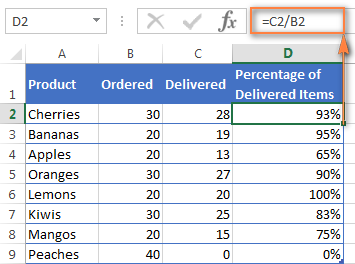## Calculating percentage of total in Excel

In fact, the above example is a particular case of calculating percentages of a total. Now, let's investigate a few more examples that will help you make quick work of calculating a percent of a total in Excel on different data sets.

#### Example 1. The total is at the end of the table in a certain cell

A very common scenario is when you have a total in a single cell at the end of a table. In this case, the percentage formula will be similar to the one we've just discussed with the only difference that a cell reference in the denominator is an absolute reference (with \$).The dollar sign fixes the reference to a given cell, so that it never changes no matter where the formula is copied.

For example, if you have some values in column B and their total in cell B10, you'd use the following formula to calculate percentages of the total: `=B2/\$B\$10`

You use a relative cell reference to cell B2 because you want it to get changed when you copy the formula to other cells of column B. But you enter \$B\$10 as an absolute cell reference because you want to leave the denominator fixed on B10 when auto-filling the formula down to row 9.

Tip. To make the denominator an absolute reference, either type the dollar sign (\$) manually or click the cell reference in the formula bar and press F4.

The screenshot below demonstrates the results returned by the formula, the Percentages of Total column is formatted as percentage with 2 decimal places showing.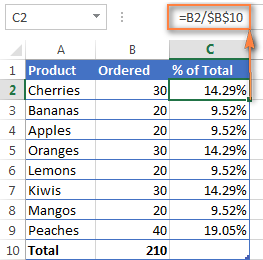#### Example 2. Parts of the total are in multiple rows

In the above example, suppose you have several rows for the same product and you want to know what part of the total is made by all orders of that particular product.

In this case, you can use the SUMIF function to add up all numbers relating to a given product first, and then divide that number by the total, like this:

`=SUMIF(range, criteria, sum_range) / total`

Given that column A contains all product names, column B lists corresponding quantities, cell E1 is the name of the product you are interested in, and the total is in cell B10, your real-life formula may look similar to this:

`=SUMIF(A2:A9 ,E1, B2:B9) / \$B\$10`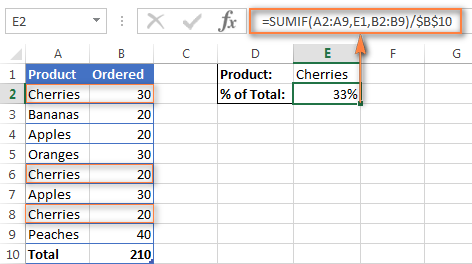Naturally, you can put the product name directly in the formula, like this:

`=SUMIF(A2:A9, "cherries", B2:B9) / \$B\$10`

If you want to find out what part of the total a few different products make, add up the results returned by several SUMIF functions, and then divide that number by the total. For example, the following formula calculates the percent of cherries and apples:

`=(SUMIF(A2:A9, "cherries", B2:B9) + SUMIF(A2:A9, "apples", B2:B9)) / \$B\$10`

## How to calculate percent change in Excel

Of all formulas for calculating percentage in Excel, a percent change formula is probably the one you would use most often.

#### Excel formula for percentage change (percentage increase / decrease)

To calculate percent change between values A and B, use the following formula:

Percent Change = (B - A) / A

When applying this formula to real data, it is important that you correctly determine which value is A and which is B. For example, yesterday you had 80 apples and how you have 100, meaning that now you have 20 apples more than before, which is 25% increase. If you had 100 apples and now you have 80, your number of apples has reduced by 20, which is 20% decrease.

Considering the above, our Excel formula for percentage change takes the following shape:

(New Value - Old Value) / Old Value

And now, let's see how you can use this percentage change formula (aka Excel percentage increase formula) in your spreadsheets.

#### Example 1. Calculating percent change between 2 columns

Suppose that you have the last month prices in column B and this month prices in column C. Then you can enter the following formula in cell D2 to calculate percentage change in your Excel sheet:

`=(C2-B2)/B2`

This percent change formula calculates the percentage increase / decrease in this month (column C) comparted to last month (column B).

After copying the formula to other rows, remember to click the Percent Style button on the ribbon to display decimals as percentages and you will get a result similar to what you see in the screenshot below. In this example, positive percentages that show percentage increase are formatted in usual black, while the negative percentages (percent decrease) are formatted in red by using the technique explained in this tip.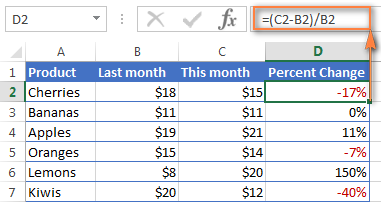#### Example 2. Calculating percentage change between rows

In case you have one column of numbers, say column C that lists weekly or monthly sales, you can calculate percentage change using this formula:

`=(C3-C2)/C2`

Where C2 is the 1st and C3 is the 2nd cell with data.

Note. Please pay attention that you should skip the first row with data and put your Excel percentage formula into the 2nd cell, which is D3 in this example.

After applying the percent format to the column with the percent change formula, you will get the following result: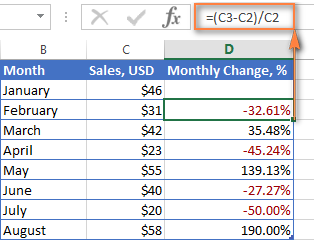If you want to calculate percentage change compared to a certain cell, then you need to fix the reference to that cell by using the absolute cell reference with the \$ sign, e.g. \$C\$2.

For instance, here is the Excel percentage change formula that calculates the percent increase / decrease for each month compared to January (C2):

`=(C3-\$C\$2)/\$C\$2`

When you drag the formula down to copy it to other cells, the absolute reference (\$C\$2) will stay the same, while the relative reference (C3) will change to C4, C5 and so on.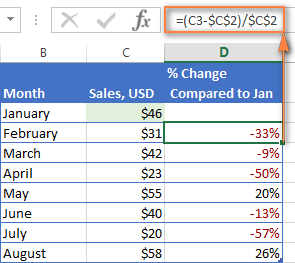## Calculating amount and total by percentage

As you've just seen, calculating percentages in Excel is easy, and so is calculating amounts and totals if you know the percentage.

#### Example 1. Calculate amount by total and percentage

Suppose you are buying a new laptop for \$950 and they charge 11% VAT on this purchase. The question is - how much do you have to pay on top of the net price? In other words, what is 11% of \$950?

The following formula will help:

Total * Percentage = Amount

Assuming that the total value is in cell A2 and percent in B2, the above formula turns into a simple `=A2*B2` and returns 104.50.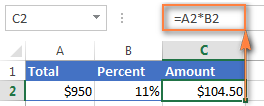Remember, when you type a number followed by the percent sign (%) in Excel, the number is interpreted as a hundredth of its value. For example, 11% is actually stored as 0.11 and Excel uses this underlying value in all formulas and calculations.

In other words, the formula `=A2*11%` is equivalent to `=A2*0.11`. Naturally, you are free to use the decimal number corresponding to the percentage directly in a formula if this works better for your worksheets.

#### Example 2. Calculating total by amount and percentage

For example, you friend offered you his old computer for \$400, which is 30% off the original price. You want to know what the original price was.

Since 30% is the discount, you deduct it from 100% first to know what percentage you actually have to pay (100% - 30% = 70%). Now you need the formula to calculate the original price, i.e. to find the number whose 70% equals 400.

The formula goes as follows:

Amount / Percentage = Total

Applied to real data, it may take any of the following shapes:

`=A2/B2` or `=A2/0.7` or `=A2/70%`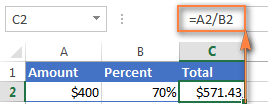## How to increase / decrease a number by percentage

The holiday season is upon us and this indicates a change in your usual weekly spendings. You may want to consider different options to find out your optimum weekly allowance.

To increase an amount by a percentage, use this formula:

= Amount * (1 + %)

For example, the formula `=A1*(1+20%)` increases the value in cell A1 by 20%.

To decrease an amount by a percentage:

= Amount * (1 - %)

For instance, the formula `=A1*(1-20%)` reduces the value in cell A1 by 20%.

In our example, if A2 is your current expenditures and B2 is the percentage you want to increase or reduce that amount by, here are the formulas you'd enter in cell C2:

Increase by percentage: `=A2*(1+B2)`

Reduce by percentage: `=A2*(1-B2)`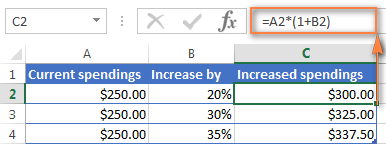#### How to increase / reduce an entire column by a percentage

Suppose, you have a column of numbers that you want to increase or reduce by a certain percent, and you want to have the updated numbers in the same column rather than add a new column with the formula.

Here are 5 quick steps to handle this task:

1. Enter all the numbers you want to increase or decrease in some column, column B in this example.
2. In an empty cell, enter one of the below formulas:

Increase by percentage: `=1+20%`

Reduce by percentage: `=1-20%`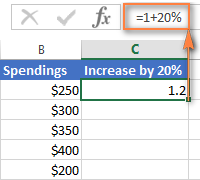Naturally, you are free to replace 20% in the above formulas with the percentage you want.

3. Select the cell with the formula (C2 in our case) and copy it by pressing Ctrl + C.
4. Select the range of cells you want to change, right click the selection and then click Paste Special…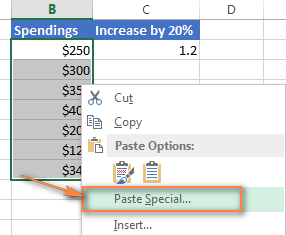5. In the Paste Special dialog window, select Values under Paste, Multiply under Operation and click OK.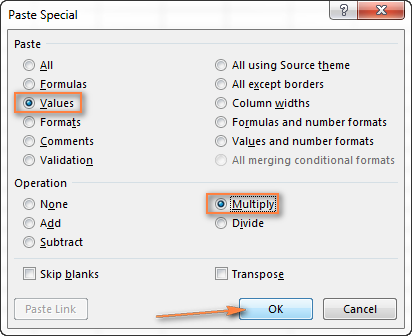And here's the result - all the numbers in column B are increased by 20%: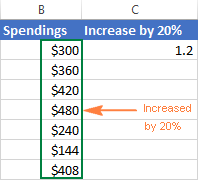In the same fashion, you can multiply or divide a column of numbers by a certain percentage. Simply enter the desired percentage in an empty cell and follow the steps above.

This is how you calculate percentage in Excel. And even if working with percentages has never been your favorite kind of math, by using these basic percentage formulas you can get Excel to do the work for you. That's all for today, thank you for reading!

### 470 Responses to "How to calculate percentage in Excel - formula examples"

1.Rita Evangelista says:

Hi,
I need to work out what percentage of people achieved a certain result range. For example, how many of a percentage achieved 99-99.90 and next group 98-98.95.
Thank you.

•Albert Kojo Garbrah says:

Please I need the answer to the same question Rita asked. Kindly help me with the formula to use. Thank you.

2.changaize says:

Hi
Formula is explained in detail and am appreciation indeed.
and in future will hope full to find more solution about excel.
Thanks and BR

3.GLORIA says:

Hi. I need to calculate percentage when the percentage number is in another cell....i hope this makes sense...

4.tommy says:

just need the formal \$ 3580.69 % of \$ 44775.39

5.Bijaya Kumar says:

For example, I have purchased a book at Rs. 495/-. Now, I want to calculate 25% off on this book then what will be the formula for this?

•sureshkumar R says:

=495*25/100

Amount you want to calculate, it has to be multiplied by the percentage then it has to be divided by 100

=495*25/100= 123.75
so you need to pay (495-123.75)=371.25

6.Kali says:

I need to find the % of tools that were scraped in each quarter, the total of tools go in the thousands and the scraps are normally under 20, how would I find the % of that?

7.G.Ali says:

I Need Price List Percentage Formula in Excel Sheet

8.Adam says:

Hello, the example I can give to explain what I need is this. I am currently at an average of 72%, how many more "100%'s" would I need to achieve an 80% average?

9.SHAIK ASLAM says:

I CANT UNDERSTAND SO CAN U
TELL ME BREIFLY

10.Rao Waqar says:

thanks and regards.

11.Fluffy says:

I need to add the GST of 15% to total

12.MOKILI says:

I am very proud with this lesson if possible i can get it in my mail, cause is very help full, Thanks a lot of this tutoriel

13.Marci says:

Hello, I need the the limit number where the higher 20% of total start.
Is it possible?

14.RJ says:

Can you please send me a formula to show the percentages for a task completed within 3, 5 or up to 30 days? I have an exact start date column and a variable date of completion. Thanks!

15.Yishak says:

Please show me Percentage calculation not by number example Male% or Female%. Thanks

16.nain says:

its good exemple

17.Shibu says:

how can i calculate, if the value is 2350/-(25%) , i need to find out the value of 75%, please give the formula.

18.Pamela Samuel says:

Hi,
Column p has total sum of rent with formula, column q has 10% fee due from column pa no formula, I would like to calculate column p by 10% to show in column R

19.jc says:

i have 6 stores each store needs 4 visits = 24
i need to find out the below:

6 stores * 4 visits need /(divided) by actual = %

20.ZUVA says:

I have to calculate the percentage increase in price.
0,0017 and 0,0033 and it comes to? 300%

21.Samji says:

B=A+5% HOW CAN I CALCULATE A IN EXCEL . I HAVE THE VALUE OF B

22.Tetteh Stephen says:

I happy on this site more examples

23.Larry Hild says:

Hello,
I greatly appreciate your tips of expertise here, as I use them to teach my students.
I wondered whether you have a formula for this example:
I have one column on an Excel 2016 sheet of dates, ranging in cells B2:B86. In B89, I'd like to reflect the amount of dates in the range; i.e., if all the cells contained a date, then obviously the percentage in B89 would be 100%. But, it looks like not ALL of the cells will have dates, so I need a formula in B89 that will reflect the percentage of dates that DID reflect in the range. Is that possible?

Thank you so very much for your help, and I'll continue to share you site with our students.

24.Salman says:

i estimated lbs. then truck went to weight stations get actual weight easy to get different between A & B weight but I want to know how to get the percentage between the estimated and the actual weight,, I did it already but its show me error mark

thank you

25.Salisu Abdullahi says:

Please i want u to help me in this.

An oil company with 7 staffs and of different salary, was given 10,000 to share among the staffs, how can i do that without in excel without using percentage.
Thank you.

26.Abdul Jabbar says:

Thank you so much! It's really helpful.

27.Archit Muchhal says:

I have a column in my excel sheet which has temperatures. I am looking to calculate for how much percentage of time do I see temperatures below "X" degC, between "X" degC and "Y" degC and above "Y" degC.
I appreciate the help. Thank you

28.Marlene says:

Hello,

How would I be able to find what missing "x" is in percentage. For example, I was given a total gross number (\$7617.00) and a certain percentage was used to come up with total taxes paid of \$571.28. How can I find out what percentage they used to come up with the \$571.28? Is there a formula I can use to figure out missing "x" value? Please help.

THank you

29.MICAH MOSBY says:

i work in a restaurant and i'm trying to track servers comps % to their sales and i want to give -5 points to them for every \$13.00 in comps for \$100 in sales. im trying to have the sales/comp table to be on one page and the points on a second page that will be printed and posted. i hope this made since, our sales are high so it would be more like 2550 in sales and 198 in comps for one server on 5 days and 1320 sales and 180 in comps for a server on 3 days so the % of that then with -5 per \$13 in comps per 100. it needs to be fair for a one day server whos going to have a low comp number vs a 5 day who will have a high comp number. if you can help or send me in the right direction i would be grateful. thank you.

30.ilarnie says:

i need the following formular were an amout equals 120%

31.MANZI JUSTIN says:

HELLO I WANT TO ASK YOU HOW TO MAKE A PERCENTAGE IN THIS FORM 12/% , BECAUSE I'M TRYING BUT I FAIL WHY I NEED TO GET THE BEST STEPS ABOUT THAT FORM.

32.Laura says:

Trying to find out how to see total percentage for a project. The project has 9 people involved. Each are tasked to complete the same tasks, but they can do at different times.
When the individual tasks that have been assigned are complete they mark it down. At any moment I can see what percentage of the tasks assigned each team member has completed. But now what i want to see is the TOTAL percentage for all the team members. So if some are at 13% complete others are at 100% ... what is the completion percentage? what formula would i need to use?

33.Mary says:

I have 23 people in my division. We have to take 10 mandatory trainings. I want to find the percentage for each person as well as the percentage for the division for each class. How do I do this?

34.Salisu says:

Please, how to calculate the value plus 5 percent of the value

•Svetlana Cheusheva (Ablebits.com Team) says:

Hi Salisu,

Assuming the value is in A1, use one of these formulas:

=A1+A1*0.05 or =A1*(1+5%)

35.John says:

I need to enter the formulas that calculate the percentage of the grand total for each type of school supply ( total cost of each item divided by the grand total) my formulas must use appropriate absolute cell references. Format the calculated values as Percentage with 0 decimal places.
Cells B(cost/unit) C(Sept.Units) D(Sept.Cost) E(% of Grand Total)
B5\$0.75 C5 29 units D5\$21.75 E5 3%

36.Chedu says:

A B C
Name percentage Result
ZENITH 55
ANUP 30

How to Analyze this data table ??

37.Gershon Tahyor says:

Thanks for the tutorial I appreciate but please send it to my email

38.INEZA serge says:

hi it may help to the people opened realy their mind clear

39.Marie says:

How do I create a negative percentage when one of my values is zero?
Target Quantity On Hand
(8,000) 0

I have an overabundance of supply based on my target (8,000 grams) and 0 grams on hand to make more product - percent to target should be negative.

40.karthik says:

sir,
what is this amount of percentage below the amont
1,475,196.00 92,791,034.00

41.Arpita Gajbhar says:

300 is total and 192 is marks then how many percentage

•sureshkumar R says:

=192/300*100

=64%

42.sureshkumar R says:

=495*25/100

Amount you want to calculate, it has to be multiplied by the percentage then it has to be divided by 100

=495*25/100= 123.75
so you need to pay (495-123.75)=371.25

43.Cheryl Porter says:

trying to put a sum in which will calculate superanuation of 9.5% of total wages for example employee was paid 52,206.10 need to calculate 9.5%

44.dipesh khadka says:

THANKS A LOT THIS VALUABLE INFORMATION.

45.R.D.Sunil says:

Hi,
I would like to calculate to know the rates by a percentage for example
1 kgs Coffee is 100/- and 1Kg Chicory 80/- and now I need to divide the rates with a formula like if I enter 1 kg marking 70% coffee and 30% Chicory I need the right answer on my excel table please help me to solve this......

46.kamran says:

Hi.
i want to give 100 marks if percentage is 85%. how can i do this in excel. Any formula, please.

47.Sabo Moses. says:

From your explanations, solutions are glaring. Thank you.

48.Tony I says:

I am using excel to keep track number of tickets sold for a h.s. reunion and their totals. That piece is done. I and am using PayPal for credit card processing they charge 2.9% I am not able to figure out that particular formula. I am keeping the total raw ticket amounts in cell D112.
I would like to take D112 * 2.9% and place the result into B114. I have tried and tried to figure out the proper formula with no luck. Can you please help me out with this. It should be a simple formula but it seems to elude me

49.Lanie Camarillo says:

How to calculate grades in percent using excel?

50.CK says:

Hi,
Thank you for this article. I may have a slightly complicated case.
Cells A1 through A3 have fixed values. Cells B1 through B3 will have different percentages. Cell B4 will have the total of Cells A1 through A3 after they have been adjusted by the corresponding percentages in Cell B1 through B3. For instance, A1 is 10, B1 is 100%. A2 is 20, B2 is 50%. A3 is 30, B3 is 0%. The expected value in B4 should be 20. What is the correct formula to use in B4, and is there a way to use ranges for this so that if I insert more rows they will automatically be captured in the formula?
Thanks very much.

•CK says:

Hi, it's me again. I just wanted to say that a friend of mine helped me out to use the SUMPRODUCT function properly. I had incorrect syntax. =SUMPRODUCT(\$A1:\$A3,B1:B3) is what should be in cell B4. Also the reason I use dollar signs in front of A1 and A3 is because when I copy this formula to column C so that I can give a different set of percentages, C4 will still reference the initial values in the A column. I hope that someone out there finds this information useful.
I also want to thank the author for using a comment section that does not require registration. I know there is a lot of abuse out there with bots, but I'm the type of person that doesn't bother posting if there's registration required.
Best regards,
CK

51.Hermes says:

Isn't the first example of apples wrong?
If I have 20 apples for 5 friends, then each should receive 20% and not 25%.
I think that formula is not suitable for this example.

•Svetlana Cheusheva (Ablebits.com Team) says:

Hi Hermes,

Suppose, you have 20 apples and you gave 5 to your friends (not to 5 friends, you just give 5 apples to someone). How much did you give, percentage wise? The answer is 25% (i.e. 5 is 25% of 20).

52.Albert Kojo Garbrah says:

Hi,
I need to work out what percentage of people achieved a certain result range. For example, how many of a percentage achieved 99-99.90 and next group 98-98.95.
Thank you.

53.Safi says:

Hi,
Can anyone help me in solving this:
The baseline for one of the indicators is 100 while the target is 20. One quarterly basis the progress is Q1 80, Q2 75 and Q3 2o, it means the target is achieved in Q3. Now how can I find the Target achieved in Numbers and the Target achieved in Percentage?
Thanks

54.Poukenkam Abonmai says:

Hi, i am using excel 2007, i am having trouble dragging down the cursor. It is not calculating or updating automatically when I drag down ( which I already put the formula on the first row/column) what i am trying to do is instead of typing manually on each and every column, I want a quick percentage calculation. I've already ticked on automatic on calculation but the formulanis still not working. The formula for series and format cell is working but not the percentage one. Someone please help.

55.Ramesh Yadav says:

Hi,
i am having two times one is incoming time second one is outgoing time
from these i need to pull out >24 and <24 data and there percentage also please healp me

56.DJ says:

Hi,
=SUM(C5)+(C5*10%) + 20% = display total value
(44.50 + 11.12)+(11.12)= 67.24
i want to add 20% on total of (C5)+(C5*10%) and direct display final total H5 i.e. 67.24
Thank you.

57.B. David says:

Holy Cow: People are getting lazy or what. Almost all the queries that are submitted can be figured out by simply reading what you wrote. Just that simple. If your in Business and can not do Basic Math, you're in the Wrong Business, because this basically is taught in grade 6 math, yes, grade 6.

58.Amutha says:

How to find the answer in excel sheet below sum
10×870-48%

59.Daisy.Daniel says:

Don't ask what others have done for you, but ask what you have done for others

60.mithun sharma says:

21-18 what is difference in percentage formula in excel

61.Walter Ayelanok says:

How do you distribute say an amount to different cost centres using percentage?

62.Samantha says:

Hello! I understand your discussion great! Super helpful. Is there a way i can make my numbers automatically round up in the same answer column? For example, i am finding 15% of 242 to = the number of records i need to pull for a review. However the answer is 36.3. Is there a way to make any answer automatically round up to the next whole number? Thanks for your help:)

•Alexander Trifuntov (Ablebits.com Team) says:

Hello Samantha!
=ROUNDUP(A1*0.15,0)

63.eugine says:

please i want the to formula calculating 12.5% of basic salary as salary tax.
and 10% of basic salary as rent allowance on Excell. let us say basic salary is as fellow s
1year-5000
2year-2000
3year-1000

•Alexander Trifuntov (Ablebits.com Team) says:

Hello Eugine!
If I understand your task correctly, the following formula should work for you:
=IF(A1=1,5000*(0.125+0.1),IF(A1>=3,1000*(0.125+0.1),2000*(0.125+0.1)))

64.W Michael Smith says:

I need to find out the % of personnel per group and then overall organizational percentage completed required 40-hours continued educational training.
I have Divisions (4 each) and under each Division is Departments (see below)
San Diego / N1 / completed CEUs / percentage completed
San Diego / N3 / completed CEUs / percentage completed
San Diego / N5 / completed CEUs / percentage completed
San Diego / N7 / completed CEUs / percentage completed
San Diego / N9 / completed CEUs / percentage completed

I have the total completed units per employee and his/her % completed
"What I need to know is N1 combined is what % completed for the year, and the same for N1, N5, N7 & N9
can someone assist?

65.Dharmendra Rana says:

Incentive (₹) Calls Target
Not Applicable >50
5₹ 50 to 100
7₹ 101 to 201
10₹ over 202
A agent is taking 300 call in a month.How much will be total money?
How to calculate incentive by formula.

66.Alexander Trifuntov (Ablebits.com Team) says:

Hello Dharmendra!
If I understand your task correctly, the following formula should work for you:

=(((A1-201)*10)+101*7+51*5)*(A1 > 201)+ ((A1-100)*7+51*5)*(A1 > 100)*(A1 <= 201)+ ((A1-49)*5)*(A1 =50)

I hope this will help, otherwise please do not hesitate to contact me anytime.

67.Michael Dye says:

How do you calculate the percentage difference in (2) prices? I have vendor A offering this material for \$1000 and vendor B is offering the same material for \$800.

•Alexander Trifuntov (Ablebits.com Team) says:

Hello Michael!
To calculate how many percent price B (800) is less than price A (1000), divide the difference between prices by price A. Multiply by 100%
(1000-800)/1000 * 100%

68.Ball Law Ram says:

I want to get 100% based on in total
example
3
37
total 40
=(3/40)*100% = 8%
=(37/40)*100% = 93%
total 101%
So it showing more than 100%. In this case, how i should do, please guide me
Thank you

69.Archie says:

Given an appraisal score in E5 and the current salary in F5, how do I calculate the new salary which could be either a)an increase by 10%,if the appraisal score is at least 75,or b)an increase by 5% if this score is greater than 60.Else,the if statement must return the text,No INCREASE.

•Alexander Trifuntov (Ablebits.com Team) says:

Hello Archie!

=IF(E5>75,F5*1.1,IF(E5>60,F5*1.05,F5))

I hope this will help

70.lalith says:

hi how to increase the value of a cell by adding some percentage
ex: F16+25%

71.Sunil Chauhan says:

I would like to know the Amount of 30% = Rs. 120

60+ professional tools for Excel